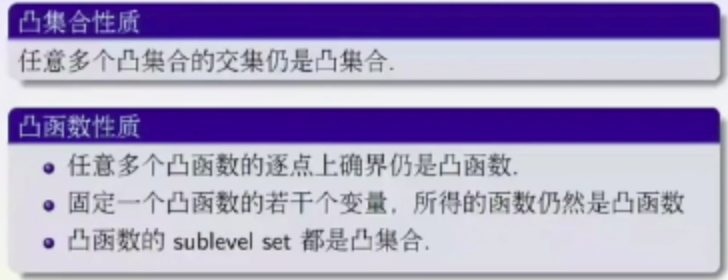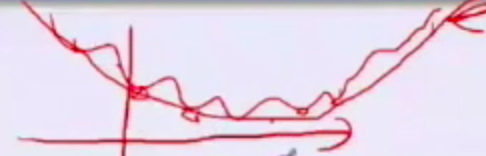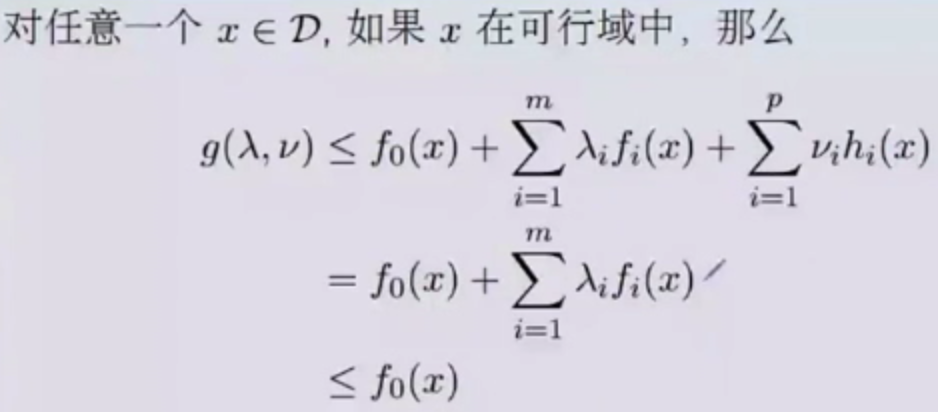# 凸优化

## 来，上概念

• 仿射集：超平面
• 凸集
• 凸函数
• (凸函数的)上境图
• (一堆点的)凸组合：重心
• (集合的)凸包
• (函数的)凸闭包：包住函数的函数，呵呵
• 下确界：凸集的支撑平面

• 上面的两点，其实还有个多点的版本。 $x=\sum_{i=1}^{k}\theta x_i,$ 条件是：$\sum_{i=1}^{k} \theta_i = 1 , x1,…,x_k \in C$ 其实这个也是定义一个凸集出来。比如说，3个点，他们在这个约束下，会变成一个三角形，一个集合中任何3个点围成一个三角，然后这些三角不断地交集，最后搞成一个大凸集，就是这种感觉，这个数学定义真的是需要想象力的。

• 一些思考： 是仿射集一定是凸集, 凸集不一定是仿射集 这两段有点反直觉啊，你想啊，直线集合更大，线段是直线的子集，直线对仿射，线段对凸集，那么按理说，凸集应该是仿射的子集把。我靠！结论正好想法啊，难怪邹博都说错了。 不过，你再细想，其实凸集更自由，而仿射要求直线（也就是线性组合，其实约束更强了），其实，不用那么纠结，凸集就是个大锅，仿射就是个平面（高维就是个线性约束），你这么想就释然了。

• 凸集、凸集合：任意两点的连线的线段上的点还在凸集中 $\lambda x_1 + (1-\lambda) x2 \in \Omega$ $\forall x_1,x_2 \in \Omega, \lambda \in (0,1)$g(x)是f(x)的凸闭包• 任意多个函数的逐点上确界仍是凸函数，就是说俩函数，绿色那个曲线，是个凸函数。

• 凸函数的sublevel set都是凸集合 这是个啥意思？## Jensen不等式

Jensen不等式 函数的组合大于组合的函数。 $\sum_{i=1}^{n} w_i f(x_i) \geq f(\sum_{i=1}^{n} w_i x_i)$ 这玩意看着很玄幻，怎么推理出来的呢？ 我们知道上境图的定义是：${ (x,y) : y\geq f(x), \forall x \in \Omega}$，也就说，(x,y)这个点是在凸集里面的。 那么就可以吧jensen不等式理解成，（$\sum_{i=1}^{n} w_i x_i, \sum_{i=1}^{n} w_i f(x_i)$ ）这个点在上境图里，注意！，把这玩意看成是一个点了，他要是在凸集的上境图里的话，那他一定是满足这个jensen不等式了，对不对？对不对？ 那爱思考的你问了，为什么丫就在凸集里面呢？ 问的好，因为你可把（$\sum_{i=1}^{n} w_i x_i, \sum_{i=1}^{n} w_i f(x_i)$ ）看作是 （$\sum_{i=1}^{n} w_i (x_i, f(x_i))$ ），这个就是条件里说的凸组合啊，凸组合的组成的这个点（重心），一定在这些点的凸包(凸包一定是凸集)里面，也就在上境图里，那么，就满足上境图的定义，就得出了结论了呀。

Jenson不等式的几何含义，就是，x是x1，x2的凸组合，那么x一定在函数的上境图里面，咳，其实就是说的这事，多直白啊，废话半天，就是这么一个直白的结论。

## 凸优化

$f_0, f_1, …, f_m$ 都得是凸函数，这样局部最优就是全局最优了。### 共轭函数#### 对偶问题$L(x,\lambda,\nu)=f_o(x)+\sum_{i=0}^{m} \lambda_i f_i(x) + \sum_{i=1}^{p} \nu_i h_i(x)$ $\lambda_i \geq 0$

$g(\lambda,\nu)=inf_{x \in D} L(x,\lambda,\nu) =$ $inf_{x \in D} ( f_o(x) + \sum_{i=0}^{m} \lambda_i f_i(x) + \sum_{i=1}^{p} \nu_i h_i(x))$ *$inf$是下确界 *$f_0, f_i$都是凸函数，$h_i$是仿射函数

$\lambda \geq 0$, 则 $g(\lambda, \nu) \leq p^*$ $p^*$ 是原问题的最优化（最小）值#### 对偶和共轭

$g(\lambda,\nu)= inf_x f_0(x) + \lambda * (Ax-b) + \nu (Cx-d)$ $=-b\lambda - d\nu + inf_x(f_o(x)+ (A\lambda + C \nu) x)$ $= -b\lambda - d\nu - f_0^* (-A\lambda - C\nu)$ 这样，我们就可以把对偶转成共轭来计算了。

（我有个问题，即使是最大了，那也不一定是原问题的最小值呀？可能很接近吧，也可能连接近都不接近吧？？？） 佩服自己，我理解的对，不一定相等的，也就是小于等于的是弱对偶，而相等的叫强对偶。

#### KKT条件

$f_i(x^* ) \leq 0$ $f_i(x^* ) \leq 0$ $h_i(x)=0$ $\lambda^* \leq 0$ 那么我们假设满足的时候，对应的最优解是$x^* , \lambda^* , \mu^*$，也就是满足这个式子： $d^* =g(\lambda^* , \mu^* )$，的时候，这仨分别对应的值。 $\leq f_o(x^* ) + \sum_{i=0}^{m} \lambda_i f_i(x^* ) + \sum_{i=1}^{p} \nu_i h_i(x^* )$ 最后一项，肯定是0，因为$h_i(x)=0$嘛。 $=f_o(x^* ) + \sum_{i=0}^{m} \lambda_i f_i(x^* )$ $\lambda^* \geq 0$ 和 $f_i(x^* ) \leq 0$，所以两者相乘肯定是小于0，所以： $\leq f_0(x^* )$ $=p^*$## 总结

• 拉格朗日函数，就是$L(x,\lambda, \nu)$
• 拉格朗日对偶函数，就是$g(\lambda, \nu)$，他是个凹函数

• 对于原问题是凸问题（就是原函数和约束函数都是凸函数),很容易就是强对偶，只要满足Slater条件（据说这个条件非常容易满足）
• 对于原问题不是凸问题的，这个就得满足KKT条件，就是那一大堆变态条件